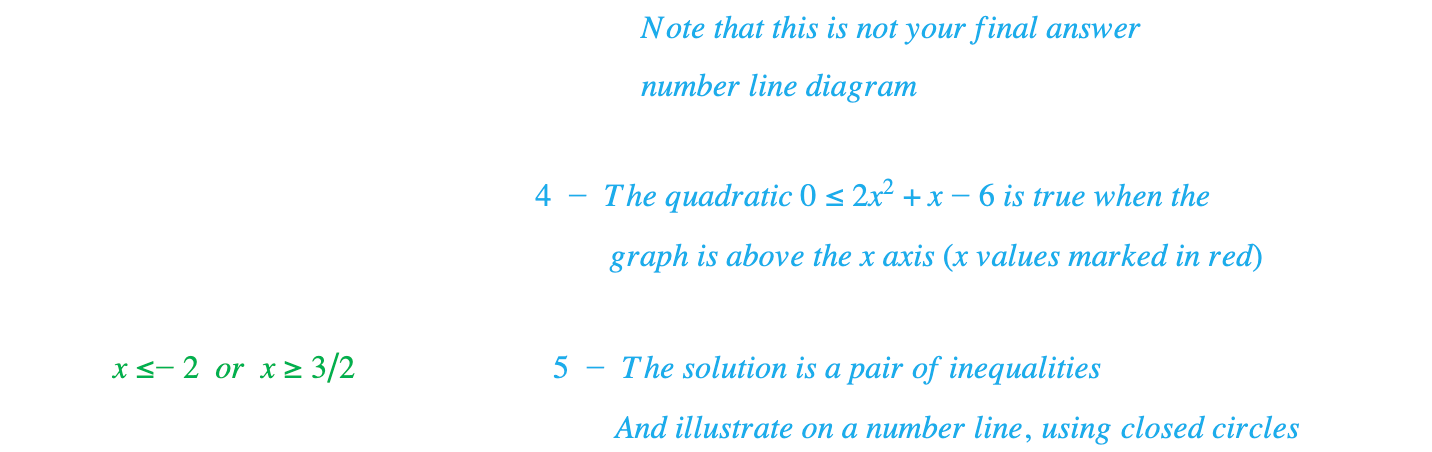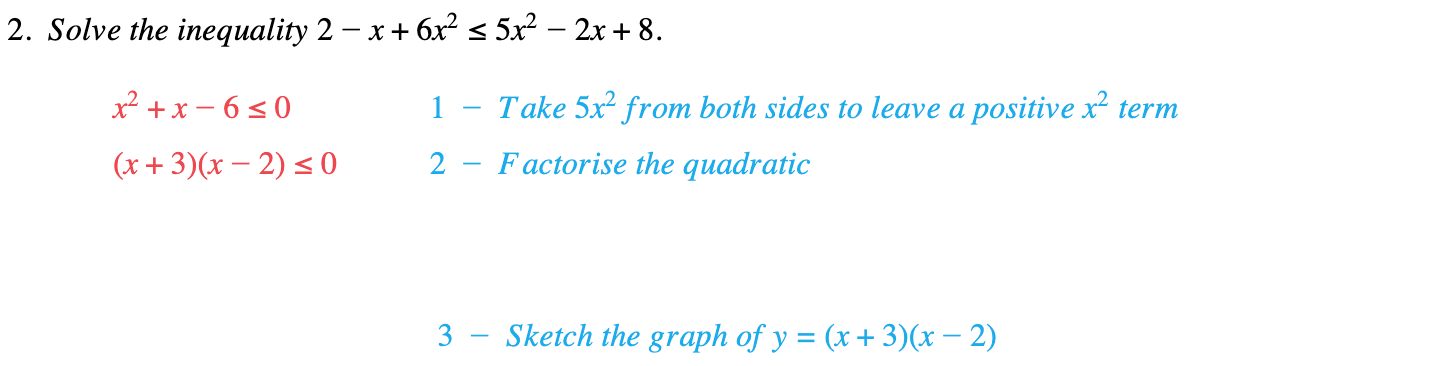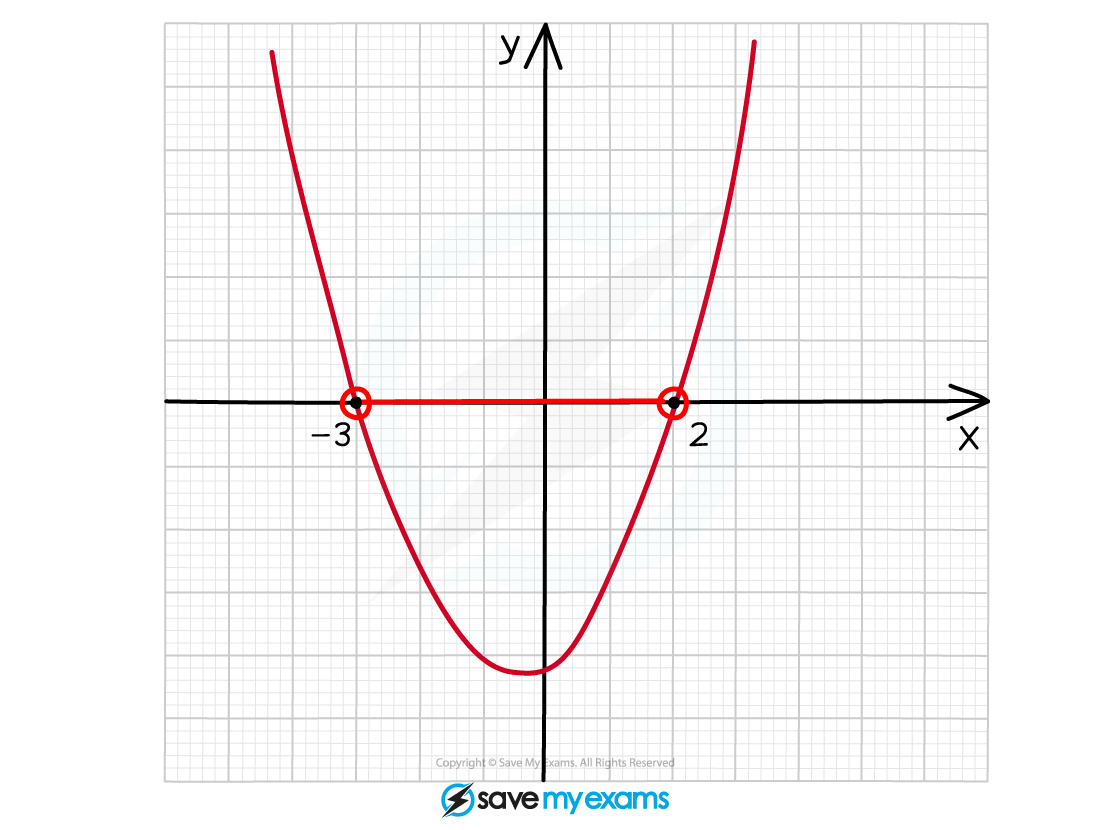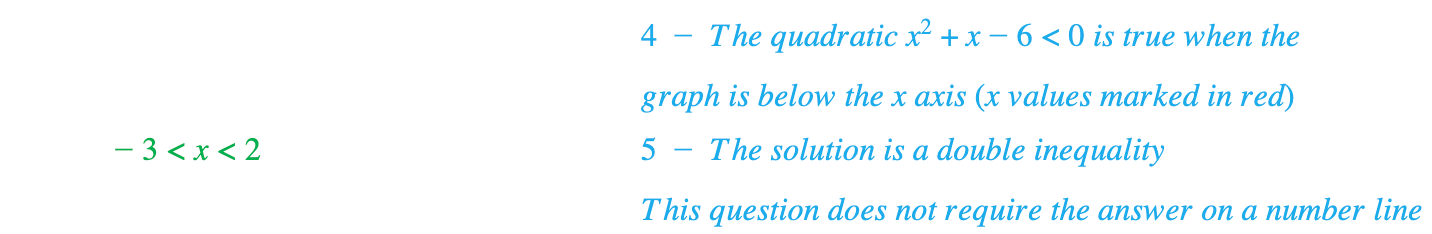# Edexcel IGCSE Maths 复习笔记 2.16.2 Solving Inequalities - Quadratic

Edexcel IGCSE Maths 复习笔记 2.16.2 Solving Inequalities - Quadratic

#### What is a quadratic inequality?

• An Inequality tells you that one expression is greater than (“>”) or less than (“<”) another
• “⩾” means “greater than or equal to”
• “⩽” means “less than or equal to”

• Quadratic Inequality has an x2 (or a y2 etc) in it
• For example, 3x2 + 4x ⩾ 7 would be read “3x2 + 4x is greater than or equal to 7”

• Solving quadratic inequalities requires you to be able to factorise quadratics so you might want to have a look at those notes first
• You also need to know how to SKETCH graphs and use Number Lines
3. SKETCH a graph of the quadratic
4. Use x-axis as a NUMBER LINE to show where the graph is above (if ≥ or >) or below (if ≤ or <) the x-axis
5. Turn the number line into a PAIR of inequalities or a DOUBLE inequality

#### Exam Tip

• When dealing with quadratic inequalities always make sure that you end up with a positive number in front of the x2
• For example, to solve the inequality 4 - 2x2 > 5x start by adding the 2x2 to (and subtracting 4 from both sides to get 0 > 2x2 + 5x -4) rather than subtracting the 5x (and getting 4 - 5x -2x2 > 0)
• Why? Because it’s much easier to factorise a quadratic when the coefficient of x2 is positive

#### Worked Example#### Worked Example#### Worked Example#### Worked Example#### Worked Example#### Worked Example#### Worked Example